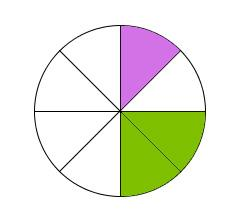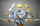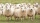# Mixed2improper

Write the mixed number as an improper fraction. 166 2/3

x =  166.6667

### Step-by-step explanation:Did you find an error or inaccuracy? Feel free to write us. Thank you!

Showing 1 comment:@bdake
I dont understand it has to addTips to related online calculators
Need help to calculate sum, simplify or multiply fractions? Try our fraction calculator.

## Related math problems and questions:

• To improper fractionChange mixed number to improper fraction a) 1 2/15 b) -2 15/17
• Fractions and mixed numerals(a) Convert the following mixed numbers to improper fractions. i. 3 5/8 ii. 7 7/6 (b) Convert the following improper fraction to mixed number. i. 13/4 ii. 78/5 (c) Simplify these fractions to their lowest terms. i. 36/42 ii. 27/45 2. evaluate following ex
• FlourKim needs 3/4 cup of flour to make 12 cookies. How much flour would she need to make 60 cookies?
• Divide 13Divide. Simplify your answer and write as an improper fraction or whole number. 14÷8/3
• Evaluate 17Evaluate 2x+6y when x=- 4/5 and y=1/3. Write your answer as a fraction or mixed number in simplest form.
• RemaindersIt is given a set of numbers { 170; 244; 299; 333; 351; 391; 423; 644 }. Divide this numbers by number 66 and determine set of remainders. As result write sum of this remainders.
• Write 2Write 791 thousandths as fraction in expanded form.
• On a farmOn a farm, there are 90 rabbits, 700 sheep, 300 cattle and 500 pigs. What percentage of the total number of animals are rabbits?
• Fraction to decimalWrite the fraction 3/22 as a decimal.What is 4 1/2+2/7-213/14?
• Divide 11Divide the product of 4 and 5/8 by 1 1/2. Write your answer as a mixed number.
• Mixed numbersRewrite mixed numbers, so the fractions have the same denominator: 5 1/5 - 2 2/3
• NumberI think the number. If I add to its third seven I get same as when to its quarter add 8. Which is the number?
• I think numberI think number.When I add 841 to it and subtract 157, I get a number that is 22 greater than 996. What number I thinking?Add two mixed fractions: 2 4/6 + 1 3/6Write a real world problem involving the multiplication of a fraction and a whole number with a product that is between 8 and 10 then solve the problemJana and David train the addition of the decimal numbers so that each of them will write a single number and these two numbers then add up. The last example was 11.11. David's number had the same number of digits before the decimal point, the Jane's numbe